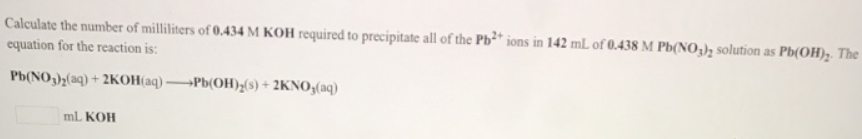# Problem: Calculate the number of milliliters of 0.434 M KOH required to precipitate all of the Pb2+ ions in 142 mL of 0.438 M Pb(NO 3)2 solution as Pb(OH)2. The equation for the reaction is: Pb(NO3)2 (aq) + 2KOH (aq) → Pb(OH) 2 (s) + 2KNO3 (aq)

###### FREE Expert Solution
91% (133 ratings)###### Problem Details

Calculate the number of milliliters of 0.434 M KOH required to precipitate all of the Pb2+ ions in 142 mL of 0.438 M Pb(NO 3)2 solution as Pb(OH)2. The equation for the reaction is:

Pb(NO3)2 (aq) + 2KOH (aq) → Pb(OH) 2 (s) + 2KNO3 (aq)